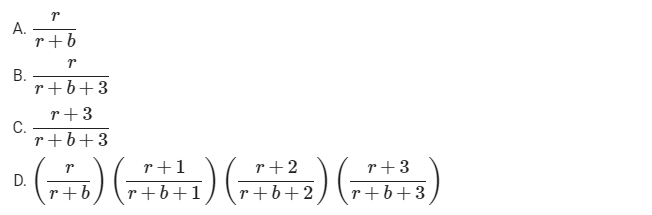Skip to content
Related Articles
GATE | GATE CS 2021 | Set 2 | Question 43
• Last Updated : 23 May, 2021

A bag has r red balls and b black balls. All balls are identical except for their colours. In a trial, a ball is randomly drawn from the bag, its colour is noted and the ball is placed back into the bag along with another ball of the same colour. Note that the number of balls in the bag will increase by one, after the trial. A sequence of four such trials is conducted. Which one of the following choices gives the probability of drawing a red ball in the fourth trial?(A) A
(B) B
(C) C
(D) D

Answer: (A)

Explanation:

Let’s Play with probability :

In 1st Attempt we pick one red ball So the probability

`= r/r+b`

In 2nd Attempt we pick one red ball (Note that now in 1st Attempt we either choose red or black balls).
So probability will be

`(r/r+b)* r+1/r+b+1 + (b/r+b) * r/r+b+1`

So even at the 10th iteration probability of choosing red ball is same.
Correct Option (A)

Attention reader! Don’t stop learning now. Learn all GATE CS concepts with Free Live Classes on our youtube channel.

My Personal Notes arrow_drop_up